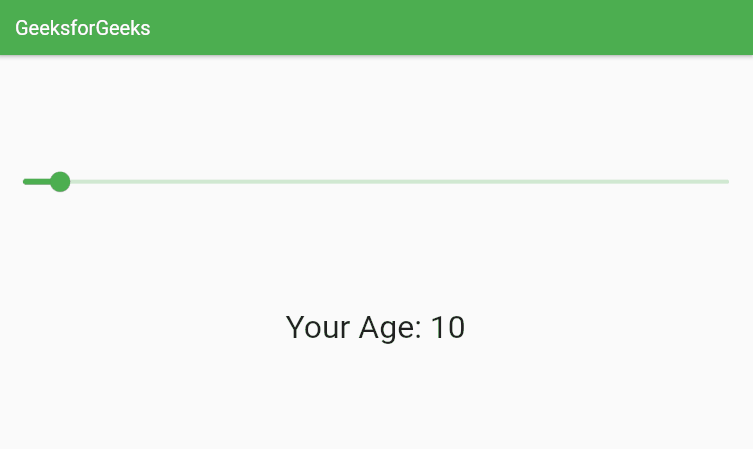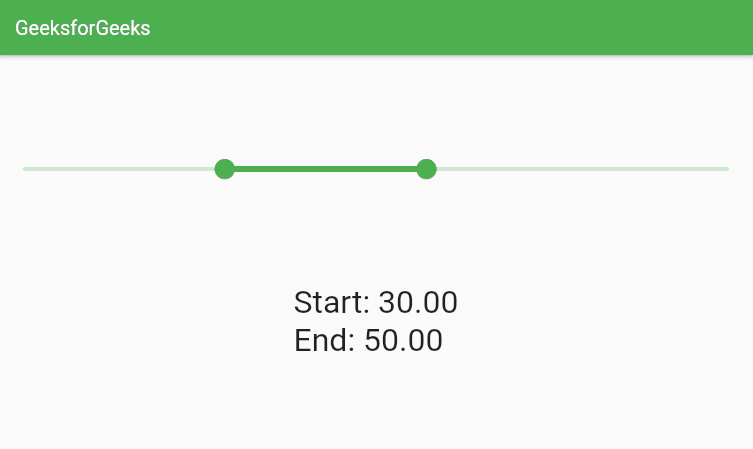Open in App
Not now

# Flutter – Slider and RangeSlide

• Last Updated : 26 Nov, 2021

A slider is a widget to select a value from a given range in the application. We can slide through the value and select the desired value from it. We don’t need to install any dependency to implement a slider.

A Range Slider is very similar to a Slider widget but instead of selecting a single value, we can select a continuous range of value from a given range.

## Implementation:

### Slider:

The slider widget can be implemented by simply using the slider widget and providing the range values. This widget takes two required parameters:

1. value: Here we need to pass the default value whenever the app is launched and should be of type double.
2. onChanged: This function is triggered whenever the slider value is changed are changed and we get a double value which we can use for further process.

Here is the syntax for Slider.

## Dart

 `Slider(``  ``value: value,``  ``onChanged: (value) {    ``  ``},``),`

### RangeSlider:

RangeSlider widget is implemented by using the widget called RangeSlider. This widget takes two required parameters:

1. values: Here we need to pass the RangeValues type of data which has a start and an end.
2. onChanged: This function is triggered whenever the range values are changed and we get a RangeValue value.

## Dart

 `RangeSlider(``  ``values: RangeValues(start, end),``  ``onChanged: (value) {},``)`

Example 1: Slider Widget

Here we have created an age selector slider. The user can slide the slider and select a value.

## Dart

 `import ``'package:flutter/material.dart'``;`` ` `class` `SliderTutorial extends StatefulWidget {``  ``const` `SliderTutorial({Key? key, required ``this``.title}) : super(key: key);``  ``final String title;``  ``@override``  ``_SliderTutorialState createState() => _SliderTutorialState();``}`` ` `class` `_SliderTutorialState extends State {``  ``int` `age = 10;``  ``@override``  ``Widget build(BuildContext context) {``    ``return` `Scaffold(``      ``appBar: AppBar(``        ``title: Text(widget.title),``      ``),``      ``body: Column(``        ``mainAxisAlignment: MainAxisAlignment.spaceEvenly,``        ``children: [``          ``Slider(``            ``label: ``"Select Age"``,``            ``value: age.toDouble(),``            ``onChanged: (value) {``              ``setState(() {``                ``age = value.toInt();``              ``});``            ``},``            ``min: 5,``            ``max: 100,``          ``),``          ``Text(``            ``"Your Age: "` `+ age.toString(),``            ``style: ``const` `TextStyle(``              ``fontSize: 32.0,``            ``),``          ``),``        ``],``      ``),``    ``);``  ``}``}`

Output:Example 2: RangeSlider Widget

In this example, we will implement the RangeSlider widget. We will need to create two double values, start and end respectively to implement the RangeSlider. Here is the code.

## Dart

 `import ``'package:flutter/material.dart'``;`` ` `class` `RangeSliderTutorial extends StatefulWidget {``  ``const` `RangeSliderTutorial({Key? key, required ``this``.title}) : super(key: key);``  ``final String title;``  ``@override``  ``_RangeSliderTutorialState createState() => _RangeSliderTutorialState();``}`` ` `class` `_RangeSliderTutorialState extends State {``  ``double` `start = 30.0;``  ``double` `end = 50.0;``  ``@override``  ``Widget build(BuildContext context) {``    ``return` `Scaffold(``      ``appBar: AppBar(``        ``title: Text(widget.title),``      ``),``      ``body: Column(``        ``mainAxisAlignment: MainAxisAlignment.spaceEvenly,``        ``children: [``          ``RangeSlider(``            ``values: RangeValues(start, end),``            ``labels: RangeLabels(start.toString(), end.toString()),``            ``onChanged: (value) {``              ``setState(() {``                ``start = value.start;``                ``end = value.end;``              ``});``            ``},``            ``min: 10.0,``            ``max: 80.0,``          ``),``          ``Text(``            ``"Start: "` `+``                ``start.toStringAsFixed(2) +``                ``"\nEnd: "` `+``                ``end.toStringAsFixed(2),``            ``style: ``const` `TextStyle(``              ``fontSize: 32.0,``            ``),``          ``),``        ``],``      ``),``    ``);``  ``}``}`

Output:My Personal Notes arrow_drop_up